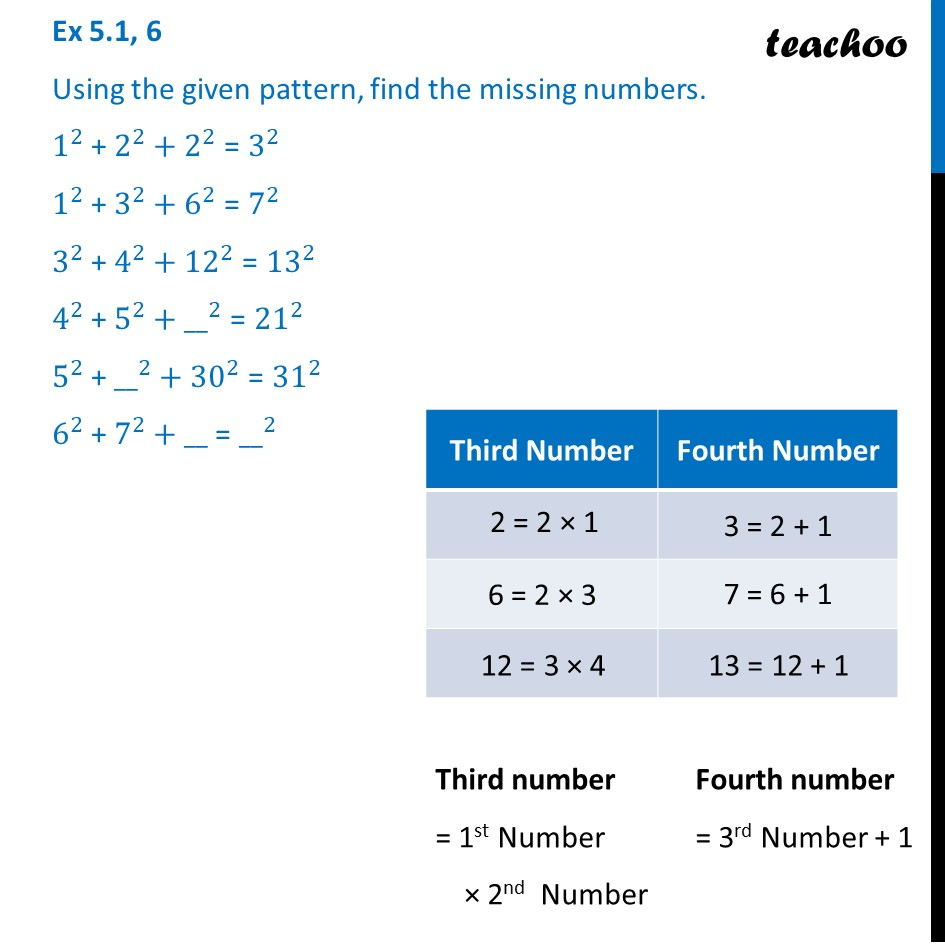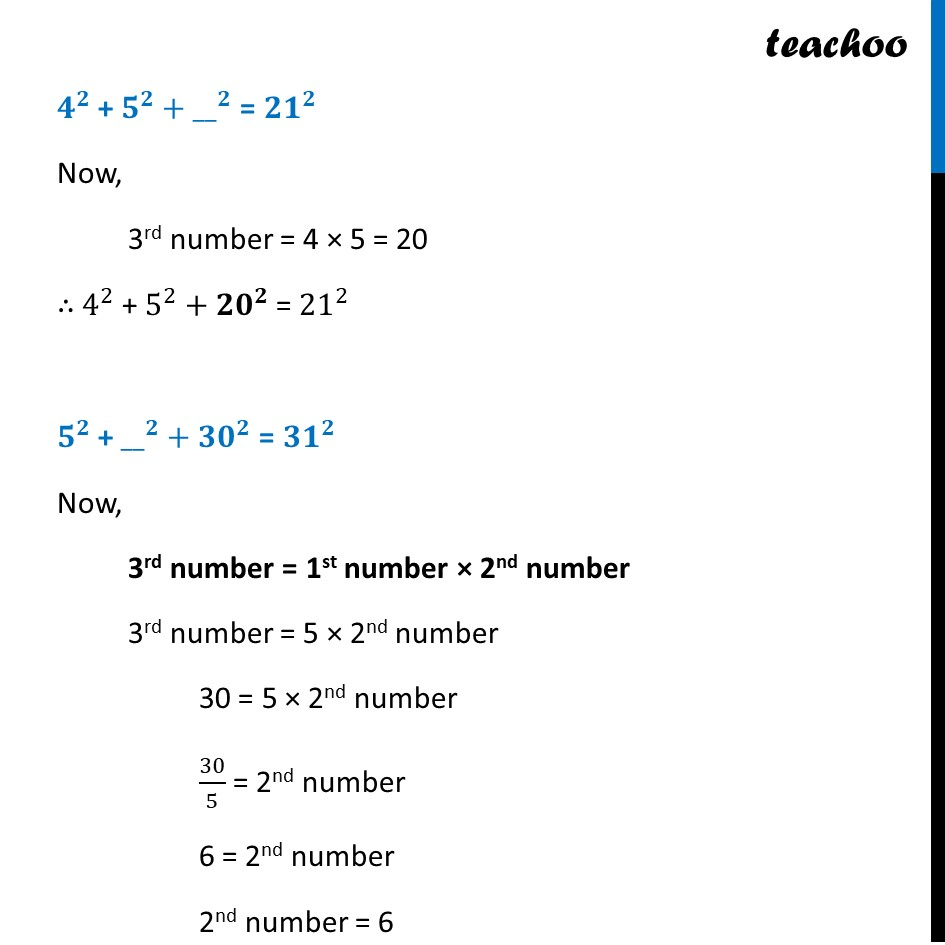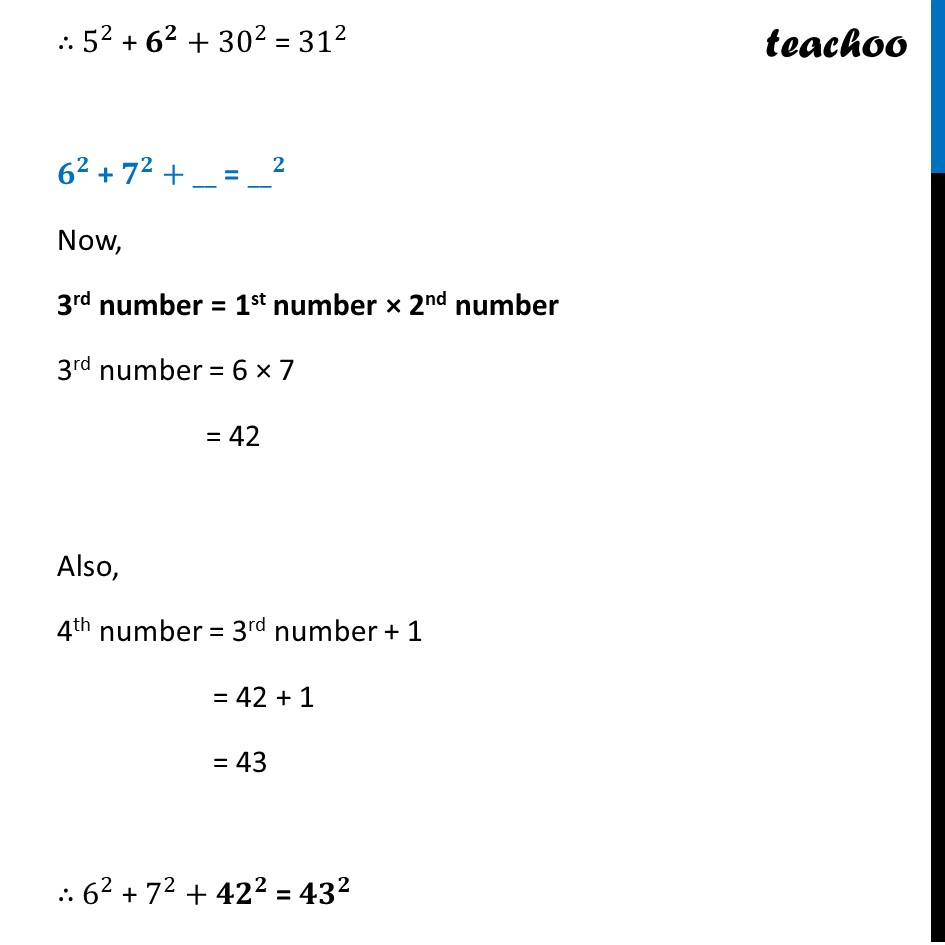Ex 5.1

Chapter 5 Class 8 Squares and Square Roots
Serial order wiseLearn in your speed, with individual attention - Teachoo Maths 1-on-1 Class

### Transcript

Ex 5.1, 6 Using the given pattern, find the missing numbers. 1^2 + 2^2+2^2 = 3^2 1^2 + 3^2+6^2 = 7^2 3^2 + 4^2+12^2 = 13^2 4^2 + 5^2+〖__〗^2 = 21^2 5^2 + 〖__〗^2+30^2 = 31^2 6^2 + 7^2+__ = 〖__〗^2Third number = 1st Number × 2nd Number Fourth number = 3rd Number + 1 𝟒^𝟐 + 𝟓^𝟐+〖__〗^𝟐 = 〖𝟐𝟏〗^𝟐 Now, 3rd number = 4 × 5 = 20 ∴ 4^2 + 5^2+〖𝟐𝟎〗^𝟐 = 21^2 𝟓^𝟐 + 〖__〗^𝟐+〖𝟑𝟎〗^𝟐 = 〖𝟑𝟏〗^𝟐 Now, 3rd number = 1st number × 2nd number 3rd number = 5 × 2nd number 30 = 5 × 2nd number 30/5 = 2nd number 6 = 2nd number 2nd number = 6 ∴ 𝟓^𝟐 + 𝟔^𝟐+〖𝟑𝟎〗^𝟐 = 〖𝟑𝟏〗^𝟐 ∴ 5^2 + 𝟔^𝟐+30^2 = 31^2 𝟔^𝟐 + 𝟕^𝟐+__ = 〖__〗^𝟐 Now, 3rd number = 1st number × 2nd number 3rd number = 6 × 7 = 42 Also, 4th number = 3rd number + 1 = 42 + 1 = 43 ∴ 6^2 + 7^2+〖𝟒𝟐〗^𝟐 = 〖𝟒𝟑〗^𝟐